Egyptian Fractions

The Egyptian scribes developed a fascinating system of numeration for fractions. To represent the sum of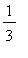andfor example, they would simply write. Actually, there's not a thing wrong with this, although it is tempting for us to go ahead and 'add' them together to get. But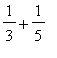is a perfectly good name for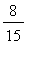, a fact we recognize when we write the equation. The problem the Egyptians had was that although they had a notation for the unit fractions, i.e., fractions of the form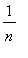, they did not have a compact notation for the general fraction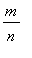. Some might say their numeration system was faulty, but that would be overly critical, since they were the first (as far as we know) to have any way of giving names to fractions.

Question : How do you suppose an Egyptian scribe would have written 3/8 ? 3/5 ?

Even though the Egyptian method of writing fractions stuck around for a long time, people were still very aware of its limitations. A good example of this is found in the Almagast , written by the Greek Mathematician, Geographer, Scientist, etc, Ptolemy in the first century AD. This book is sometimes referred to as the first trig book because it contains tables of chords for the equivalents of the sine and cosine function for use in astronomical calculations. Ptolemy
explains that he is using the Babylonian method of writing fractions rather than the Egyptian method because of the embarrassments that the Egyptian method often cause.

Much of what we know about Egyptian fractions has been inferred from the
Rhind papyrus , written by the scribe Ahmes around 1650 BC. This book consists mainly of 84 word problems of a diverse nature, plus a few tables to aid the young scriblets that Ahmes had charge of with their calculations. Generally speaking, Ahmes seemed to be happy to write the answer to a problem as a whole number plus a sum of unit fractions. He did not use any unit fraction more than once in the answer.

Problem Establish the following algebraic identity: For any n except 0,.

With this identity, you can see that a fraction can always be written in lots of different ways.
The largest table in the Rhind Papyrus is the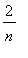table, where Ahmes gives decompositions of these fractions into sums of unit fractions. Most of the entries in the table come from the decomposition you get by multiplying the above identity on both sides by two. Thus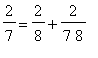=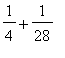.
Maple acts very naturally with Egyptian fractions. It simply adds them up and reduces to lowest terms. Thus if you enter, the output will be. We have to do something to keep a record of the decomposition, and the simplest way to do that is to make it into a list. Thus an egyptian fraction forwould be

> ef := [1/3,1/5];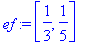>

In order to convert to the usual form we could type

> convert(ef,`+`);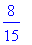>

Note: the quotes around the + are backquotes ` not forward '.

One thing that would be nice to do is take an egyptian fraction and print out an equation whose left hand side is the usual form of the fraction and whose right hand side is the fraction written as a sum of unit fractions. Because Maple is so intent on writing fractions in the usual form, we must play a trick on it. First we will convert each of the unit fractions in the egyptian fraction to a string. This can be done using the Maple word
map , like so

> ef2 := map(convert,ef,symbol);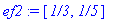Now we can write the desired equation.

> convert(ef,`+`)=convert(ef2,`+`);We will define some Maple words fibo , symbolize , and activate below which automate the process of decomposing fractions into sums of unit fractions and displaying these.

Fibonnaci's theorem

The Egyptian system of writing fractions as sums of unit fractions stuck around even after much more efficient systems were developed. Fibonnaci was aware of the system in 1200 AD and included in his book Liber Abaci a method for writing any fraction as a sum of unit fractions. His method was very natural:

Fibonnaci's method : Take the fraction you wish to express in the Egyptian manner and subtract from it the largest unit fraction which is not larger than it. If the remainder is not itself a unit fraction, then repeat the process on the remainder. Continue this until the remainder is a unit fraction.

For example, suppose

> a := 4/5 ;The largest unit fraction not larger than it is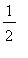. Subtract it.

> a := a - 1/2;>

The largest unit fraction not larger than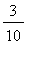is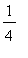. Subtract that.

> a := a - 1/4;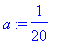>

Fibonnaci's method leads to the decomposition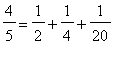.

It is not clear whether Fibonnaci had a proof that his method always worked, but a proof that it does always work can be fashioned from the following lemma.

Lemma Let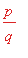be any fraction which is not a unit fraction. Letbe the largest unit fraction less than or equal to. Then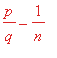is a fractionwith r < p .

Proof The argument for the lemma is simple. First write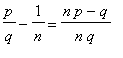. Now if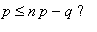, then adding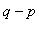to both sides of the inequality gives that. But then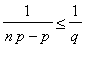. Now multiply both sides by p and get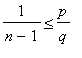. Sinceis not a unit fraction, but is less than 1, we have that n > 2, andis a unit fraction larger thanwhich is smaller than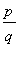. This contradicts the choice of n, and we conclude that. qed .

Theorem Fibbonaci's method works for all fractions.

Proof Starting withand subtractingwe get a smaller fraction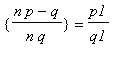. Further,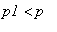by the Lemma. If p1 = 1, we are done. If not, then it will be after no more thanmore applications of the Lemma. qed .

With just a little more work, we can modify Fibbonaci's method to get a representation of any rational number as a sum of distinct unit fractions, each smaller than a given unit fraction.

Theorem Given any (positive) rational numberand any unit fraction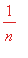,can be written as a sum of distinct unit fractions smaller than.

Proof. Since the series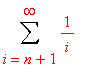diverges, there is a positive integer k so thatand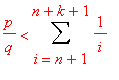. If the first inequality is an equality, thenhas been written in the required manner. If the first inequality is strict, then the remainder is a fractionwhich is less than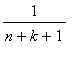. Consequently, Fibonacci's method can be used from here on to express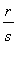as a sum of distinct unit fractions which are all distinct from the consecutive ones already used. qed .

A Maple word to carry out (the modified) Fibonnaci method.

Here is the definition of a Maple word fibo which implements Fibonnaci's method for decomposing a fraction into a sum of unit fractions. It uses two Maple words in its definition, numer , which gives the numerator of its argument, and trunc , which returns the integer part of its argument. It includes the modification established above which guarantees a decomposition into a sum of unit fractionswith n > m.

> fibo := proc(x,m)
local z,l,i,n,k;
k := m+1;
z := x;
l := NULL;
for i while 1 < numer(z) or not l = NULL and z = l[-1]
do
n := max(k,trunc(1/z)+1);
k := k+1;
z := z-1/n;
l := l,1/n;
od;
l := [l,z];
end:

To get a decomposition of 5/23 into unit fractions, enter

> ef := fibo(5/23,1);We want to write the fraction as a sum, but simply converting the list to the type `+` doesn't work because Maple adds the fractions together.

> convert(ef,`+`);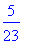We can convert the numerator and denominator of the fraction to the type symbol. Then the fractions will not combine when they are added. Symbolize takes the output from fibo and outputs a sum of symbolic fractions.

> hastype(ef,list);> symbolize := proc(frac,k)
local i,fracs;
fracs := frac;
if not hastype(fracs,list) then
fracs := fibo(frac,k) fi;
convert([seq(convert(numer(fracs[i]),symbol)/
convert(denom(fracs[i]),symbol),
i=1..nops(fracs))],`+`) end:

> symbolize(fibo(2/301,1),1);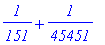It is necessary to be able to convert an eqyptian fraction from the inert form to the active form. That's what activate does.

> activate := proc(frac)
local n,m,i,j,k,bill,sam,nu,de,ans;
ans := 0;
if hastype(frac,`+`) then
for k from 1 to nops(frac) do
n := convert(numer(op(k,frac)),bytes);
m := convert(denom(op(k,frac)),bytes);
bill := [seq(48,i=1..nops(n))];
sam := [seq(48,i=1..nops(m))];
n := n - bill;
nu := convert([seq(n[nops(bill)-i]*10^i,i=0..nops(bill)-1)],`+`);
m := m - sam;
de := convert([seq(m[nops(sam)-i]*10^i,i=0..nops(sam)-1)],`+`);
ans := ans+nu/de;
od;
else
n := convert(numer( frac ),bytes);
m := convert(denom( frac ),bytes);
bill := [seq(48,i=1..nops(n))];
sam := [seq(48,i=1..nops(m))];
n := n - bill;
nu := convert([seq(n[nops(bill)-i]*10^i,i=0..nops(bill)-1)],`+`);
m := m - sam;
de := convert([seq(m[nops(sam)-i]*10^i,i=0..nops(sam)-1)],`+`);
ans := ans+nu/de;
fi;
ans;
end:

> activate(symbolize(2/1));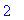> `&ea` := (fr1,fr2) -> symbolize(activate(fr1)+activate(fr2),1);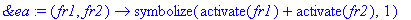> f1 := symbolize(1/6,1);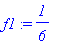> f2 := symbolize(1/5,1);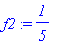> f3 :=f1 &ea f2;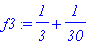> activate(f3);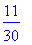> b:=fibo(9/10,1);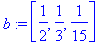> symbolize(b,1);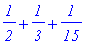> symbolize(13/10,1) ;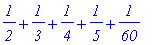>

Investigate these problems. Use fibo .

1.Print out decompositions of the fractions with an odd numerator and a denominator of 100.

2. Find a fraction which fibo decomposes into the sum of six unit fractions.

3. Find a fraction which fibo decomposes into the sum of eight unit fractions.

4. Show that there are infinitely many ways to write a given fraction as a sum of unit fractions.